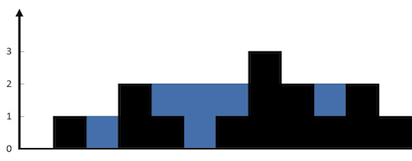# 记录leecode刷题思路

## 39.组合总数

39. 组合总和 - 力扣（LeetCode）

candidates 中的 同一个 数字可以 无限制重复被选取 。如果至少一个数字的被选数量不同，则两种组合是不同的。

2 和 3 可以形成一组候选，2 + 2 + 3 = 7 。注意 2 可以使用多次。
7 也是一个候选， 7 = 7 。

• 将数组，大集合（存储每个可能结果），小集合（存储每个可能数目），目标值，位置。
• i= 0开始，小集合放入当期数组中的值，并用目标值减去数组中的值
• 如果目标值小于0说明该不行，小集合退出该值
• 如果为0则将小集合放入大集合，但是这是有个问题，必须负责小集合的值存入，小集合中的值改变了，大集合中存入小集合的值也会变
• i<数组长度 继续进行，否则结束
class Solution {
public List<List<Integer>> combinationSum(int[] candidates, int target) {
List<List<Integer>> list = new ArrayList<List<Integer>>();
List<Integer> temp =  new ArrayList<Integer>();
Arrays.sort(candidates);
find(candidates,target,list,temp,0);
return list;
}
public void find(int[] candidates , int target, List<List<Integer>> list, List<Integer> temp, int num){
if(target< 0) return ;
if(target == 0){
return ;
}
for(int i = num; i< candidates.length ;i++){
if(target<0) break;
find(candidates,target-candidates[i],list,temp,i);
temp.remove(temp.size()-1);
}
}
}


## 42.接雨水

42. 接雨水 - 力扣（LeetCode）• 暴力破解：

• 对于每个点都需要求取左侧最大与右侧最大，这样势必会造成浪费，因此这种有重复值计算的方法很容易想到动态规划的方式。
• 双向链表：

• 定义一个左侧最大、左侧点位置、右侧最大、右侧点位置，那么双向链表只需要对两侧判断之前的最大值并且不断更新即可。
class Solution {
public int trap(int[] height) {
int count = 0 ;
int left = 0;
int right = height.length-1;
int leftHeight = height[left];
int rightHeight = height[right];
while(left<=right){
if(leftHeight<rightHeight){
leftHeight =  Math.max(leftHeight,height[left]);
count = count + Math.max(leftHeight-height[left],0);
left++;
}else{
rightHeight = Math.max(rightHeight,height[right]);
count = count + Math.max(rightHeight-height[right],0);
right--;
}
}
return count;

}
}

• 找到最高点，分别从两端往最高点走
• 走左边的时候定义一个左侧相对最大temp，如果遍历左侧的点大于temp，那么修改temp的值，如果小于则表示可以存水，temp-height(i)
• 右侧同理。

## 46.全排列

class Solution {
public List<List<Integer>> permute(int[] nums) {
List<List<Integer>> list =  new ArrayList();
List<Integer> tempList = new ArrayList();
getAll(nums,list,tempList);
return list;
}
public void getAll(int[] nums,List<List<Integer>> list,List<Integer> tempList){
if(tempList.size() == nums.length){
return ;
}
for(int i =0 ;i < nums.length;i++){
boolean isConrains = tempList.contains(nums[i]);
if(!isConrains){
getAll(nums,list,tempList);
tempList.remove(tempList.size()-1);
}
}
return;
}
}


## 48.旋转图像

#### 48. 旋转图像

class Solution {
public void rotate(int[][] matrix) {
int[][] b = new int[matrix.length][matrix.length];
for (int i = 0; i < matrix.length; i++) {
int[] a = matrix[i];
for (int j = 0 ; j < a.length ; j++){
b[i][j] = matrix[i][j];
}
}
for(int i=0;i<b.length;i++){
int[] a  = b[i];
for (int j=0;j<a.length;j++){
matrix[i][j] = b[a.length-j-1][i];
}

}
}
}

posted @ 2022-06-20 08:47  zqy123  阅读(7)  评论(0编辑  收藏  举报